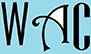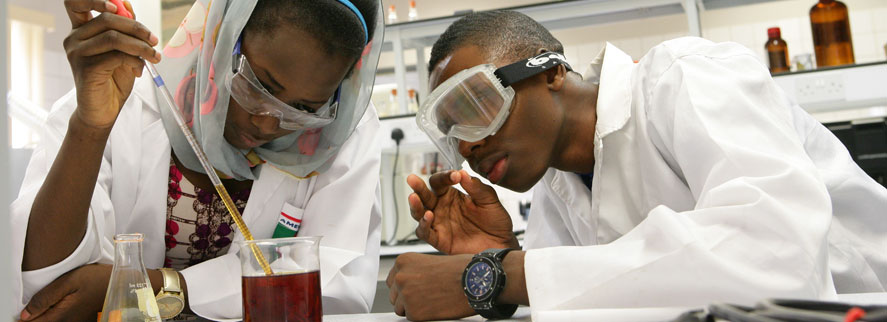## Algebra Question for Title 1 Schools: Find Original Price Question?

4) The sales price of a car is \$12,590, which is 20% of original price, what is the original price?

20% = 20/100 = 0.2

x – 0.2x = 12590 (x is the original price and we subtract 20% discount of the original price from the original price.  Keep in mid that of = multiply.

x – 0.2x = 12590

0.8x = 12590

you have to make “x” subject of formula by moving “0.8” to the other side. When you go to the other side, addition becomes subtraction, multiplication becomes division, and vice versa.

x = 12590/0.8 (0.8 was a multiplication at the other end)

15,737.50

So the original prices as \$15,737.50